# Creating plots¶

This section continues showing how to do different types of plots.

## Import modules¶

```# running locally, if you pip install then you just have to import the module
%matplotlib inline
from pandas.core.frame import DataFrame
import numpy as np
import pandas as pd
from mutagenesis_visualization.main.demo.demo_objects import DemoObjects
DEMO_OBJECTS:DemoObjects = DemoObjects()
hras_rbd = DEMO_OBJECTS.hras_rbd
hras_gapgef = DEMO_OBJECTS.hras_gapgef
```

## Histogram, scatter and more¶

Classes reviewed in this section:

There are different tools to analyze the data. The package can plot the kernel density estimation (`object.kernel`). There is the option to fit other functions to the data (see Implementation for more). You could also only plot a histogram (`object.histogram`). For the histograms, we can select to plot only the single nucleotide variants (SNVs) or the non-SNVs. In the example, it actually changes the shape of the population. Non-SNVs are more sensitive to mutations than SNVs because there is a higher proportion of non-conservative amino acid replacements.

```# Plot kernel dist using sns.distplot.
hras_rbd.kernel(
title='H-Ras 2-166', xscale=[-2, 1]
)

# Plot histogram of SNVs
hras_rbd.histogram(
population='SNV', title='H-Ras 2-166 SNV', xscale=[-2, 1]
)

# Plot histogram of non-SNVs
hras_rbd.histogram(
population='nonSNV',
title='H-Ras 2-166 non-SNV',
xscale=[-2, 1],
)
```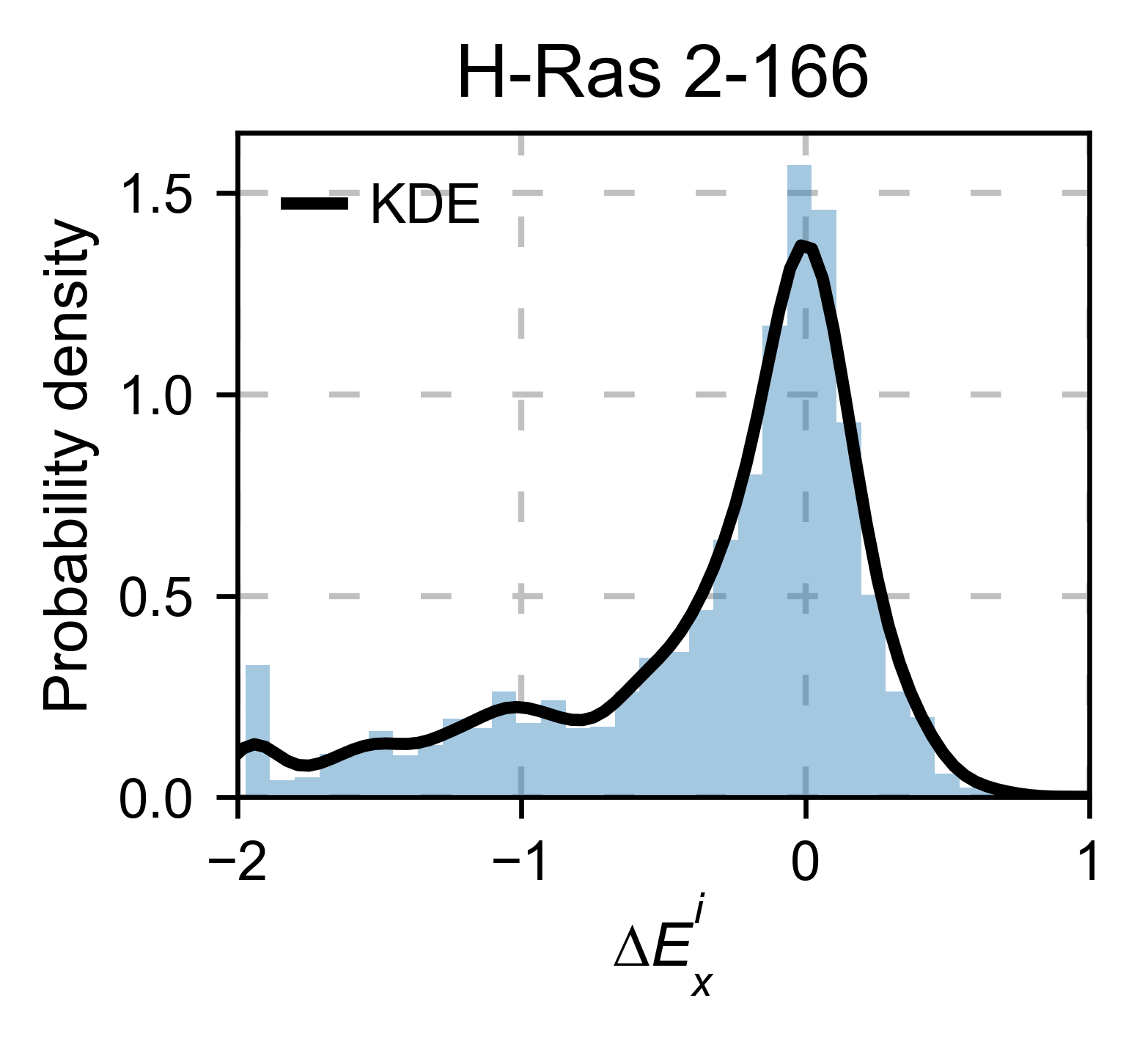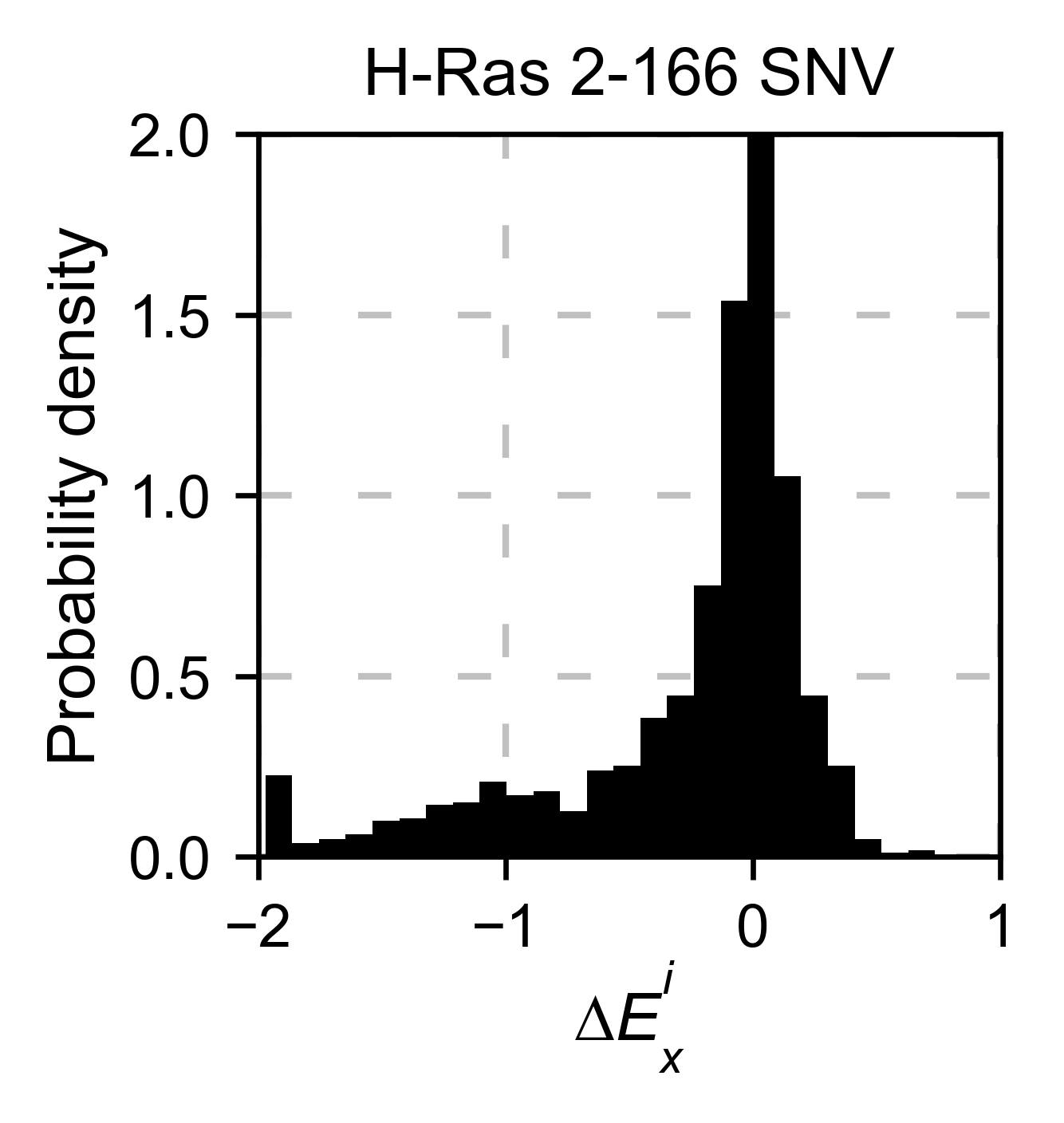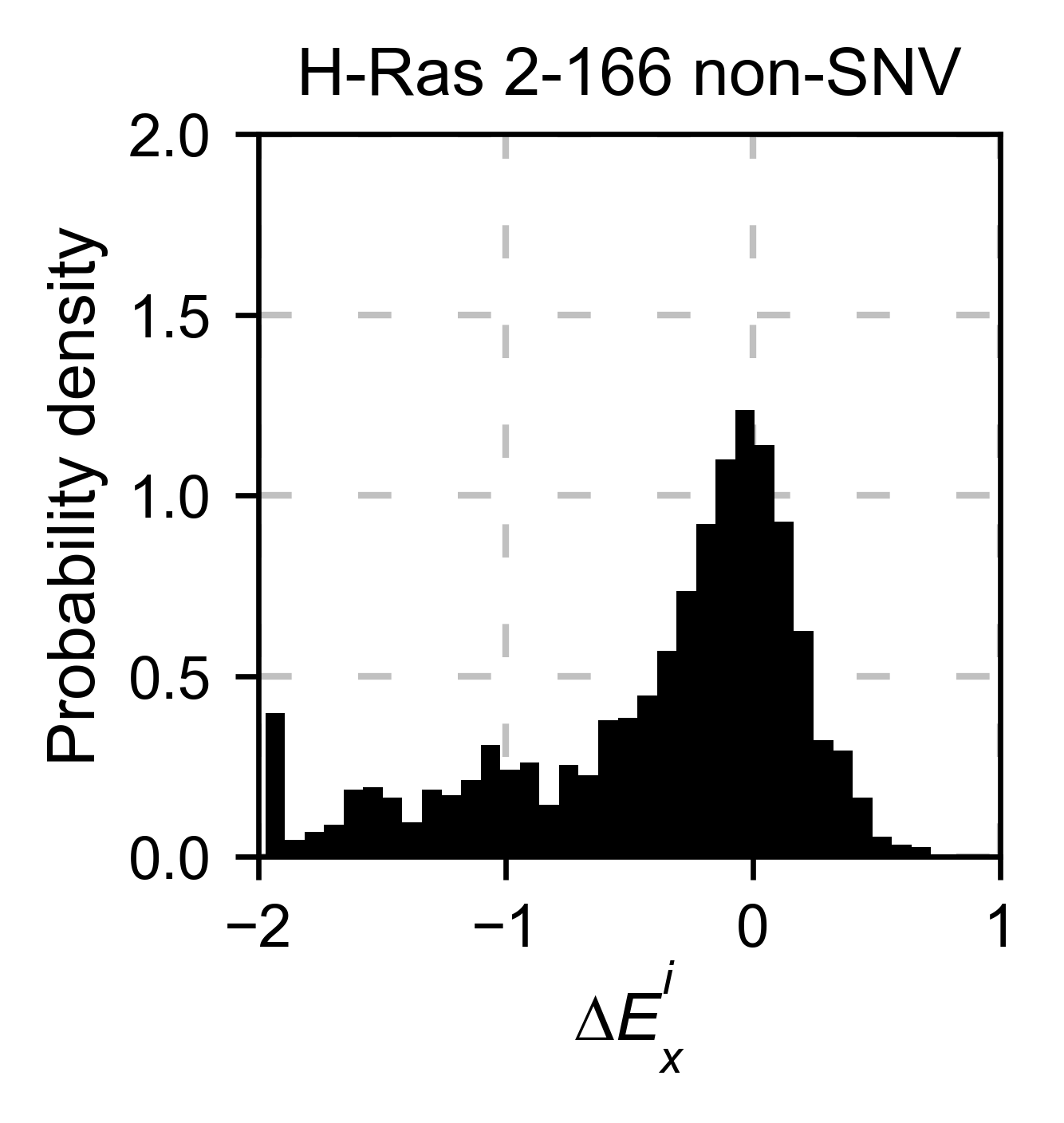If you have multiple datasets and want to compare them, you can do it with the method `object.scatter`. We give the option to do the comparison at a mutation by mutation level `mode=pointmutant`, or at a position level `mode=mean`.

```# Plot a scatter plot of each mutation
hras_rbd.scatter(
hras_gapgef,
title='Individual mutations',
mode='pointmutant',
xscale=(-2.5, 1.5),
yscale=(-2.5, 1.5),
x_label='H-Ras Unregulated',
y_label='H-Ras Regulated',
)

# Plot a scatter plot of the mean position
hras_rbd.scatter(
hras_gapgef,
title='Positional average',
mode='mean',
xscale=(-2, 1),
yscale=(-2, 1),
x_label='H-Ras Unregulated',
y_label='H-Ras Regulated',
)
```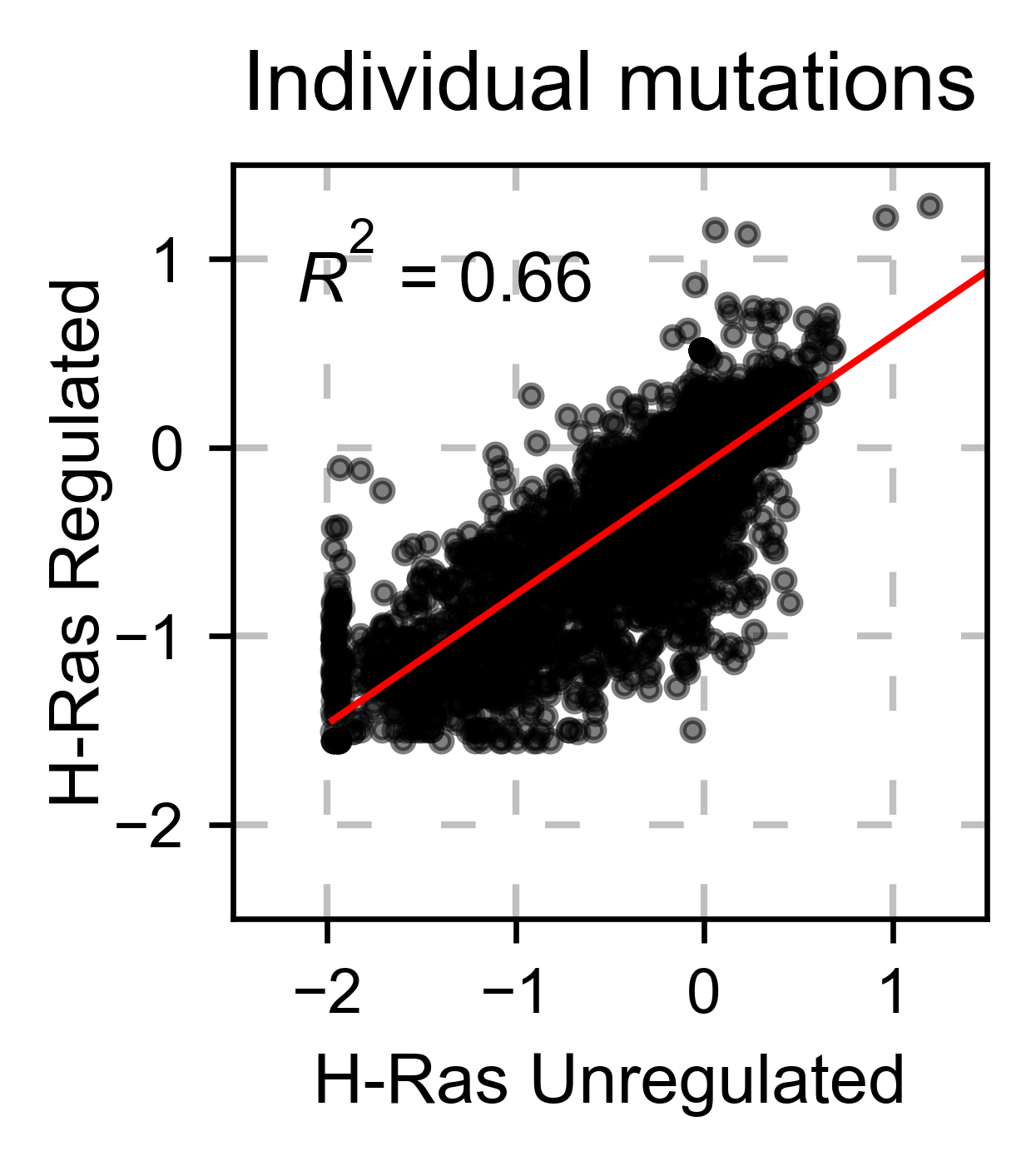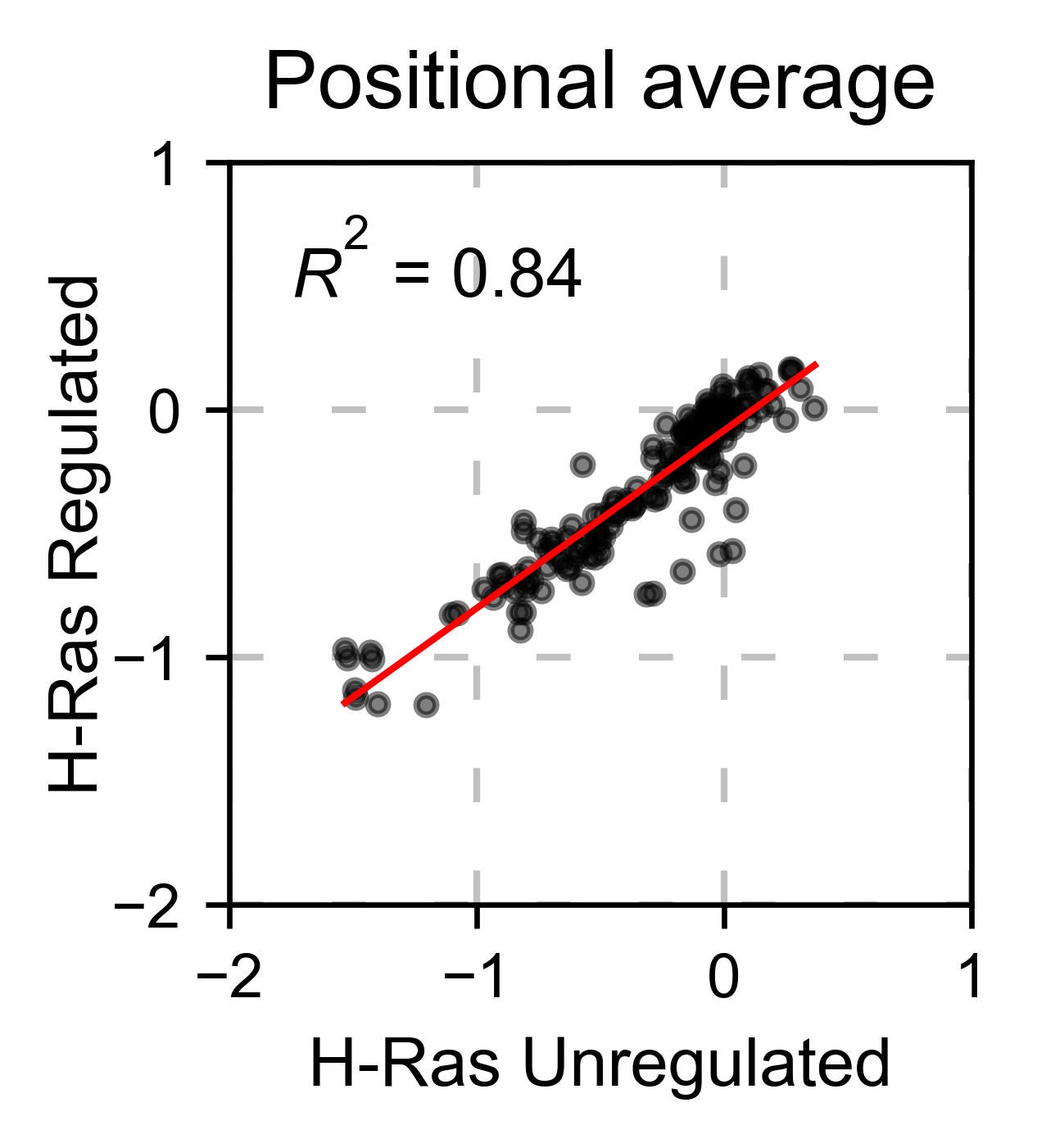If you are comparing two homologs/paralogs, you can evaluate what happens when mutating every site that differs between the two proteins to the identity in the other second protein. (ie K4A and A4K)

```# here map the residues that are different between the two proteins
map_sequence_changes = [(1, 1), (5, 5), (56, 56), (122, 123)]
#^ same residue             #^ the residue 122 of the first protein matches the 123 rd of the second protein
# ancestralras_rbd does not exist yet, so this cell wont run
hras_rbd.sequence_differences(ancestralras_rbd, map_sequence_changes)
```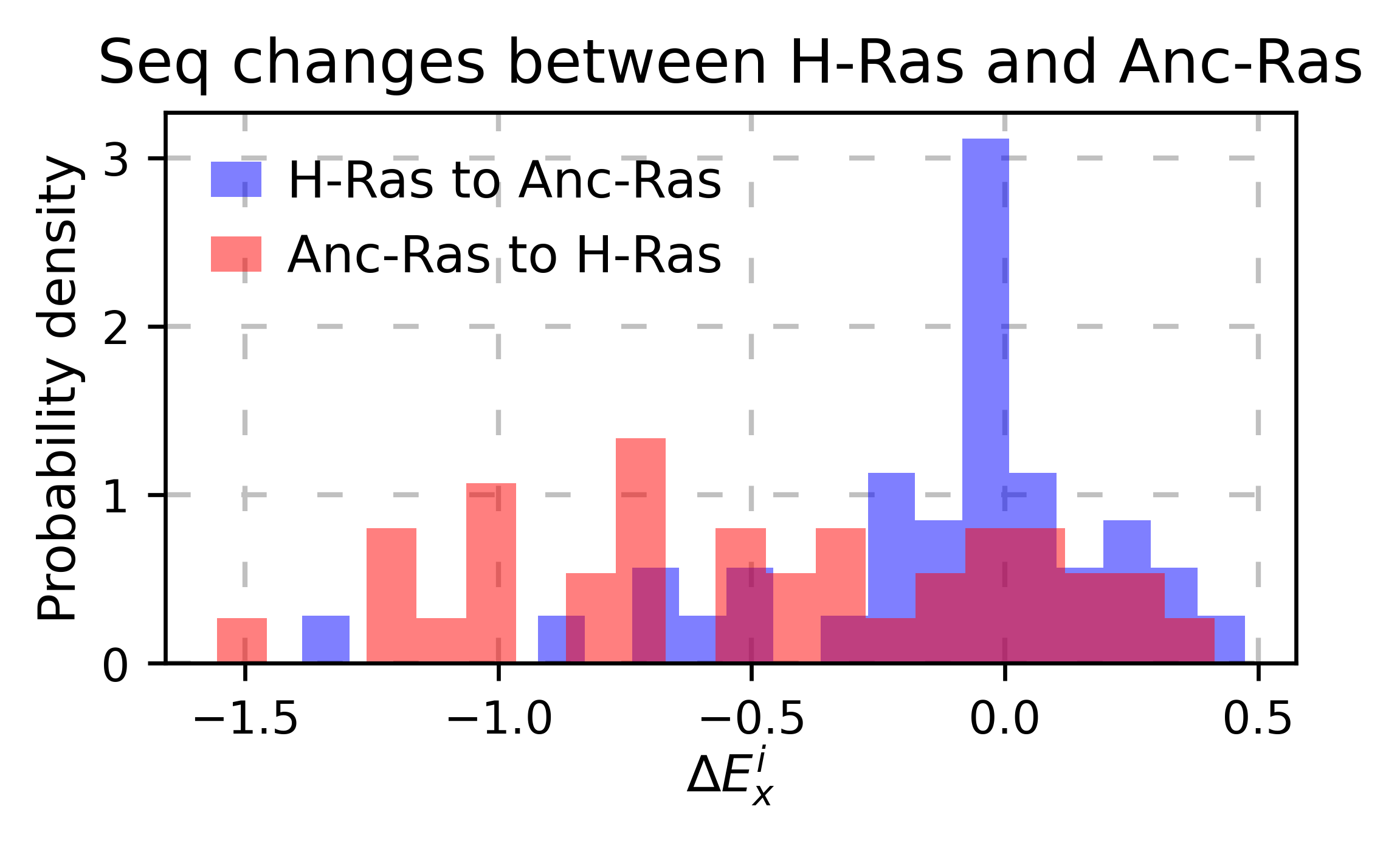The method `object.rank` sorts each mutation (or position) by its enrichment score.

```hras_rbd.rank(mode='pointmutant', outdf=True, title='Rank of mutations')
```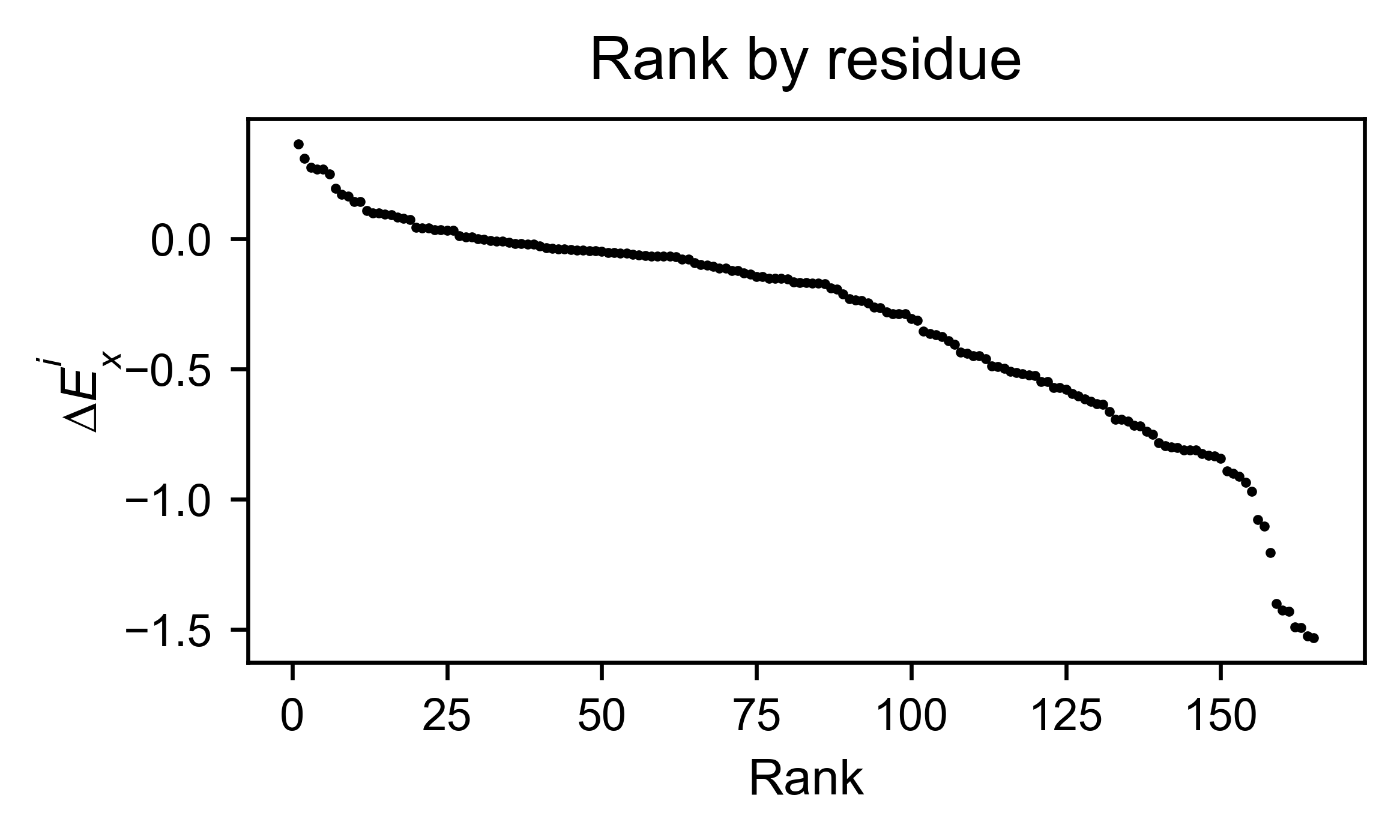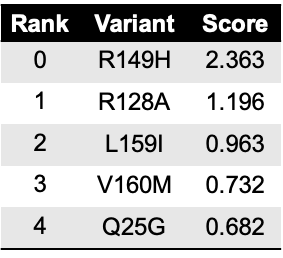The method `object.cumulative` draws a cumulative plot that sums the mean enrichment score of every position. This plot is useful to determine if the sensitivity to mutations is constant throughout the protein or not. In the example, we see that the cumulative function follows the x=y line, suggestion a homogeneous mutational tolerance.

```# Cumulative plot
hras_rbd.cumulative(mode='all', title='Cumulative Score')
```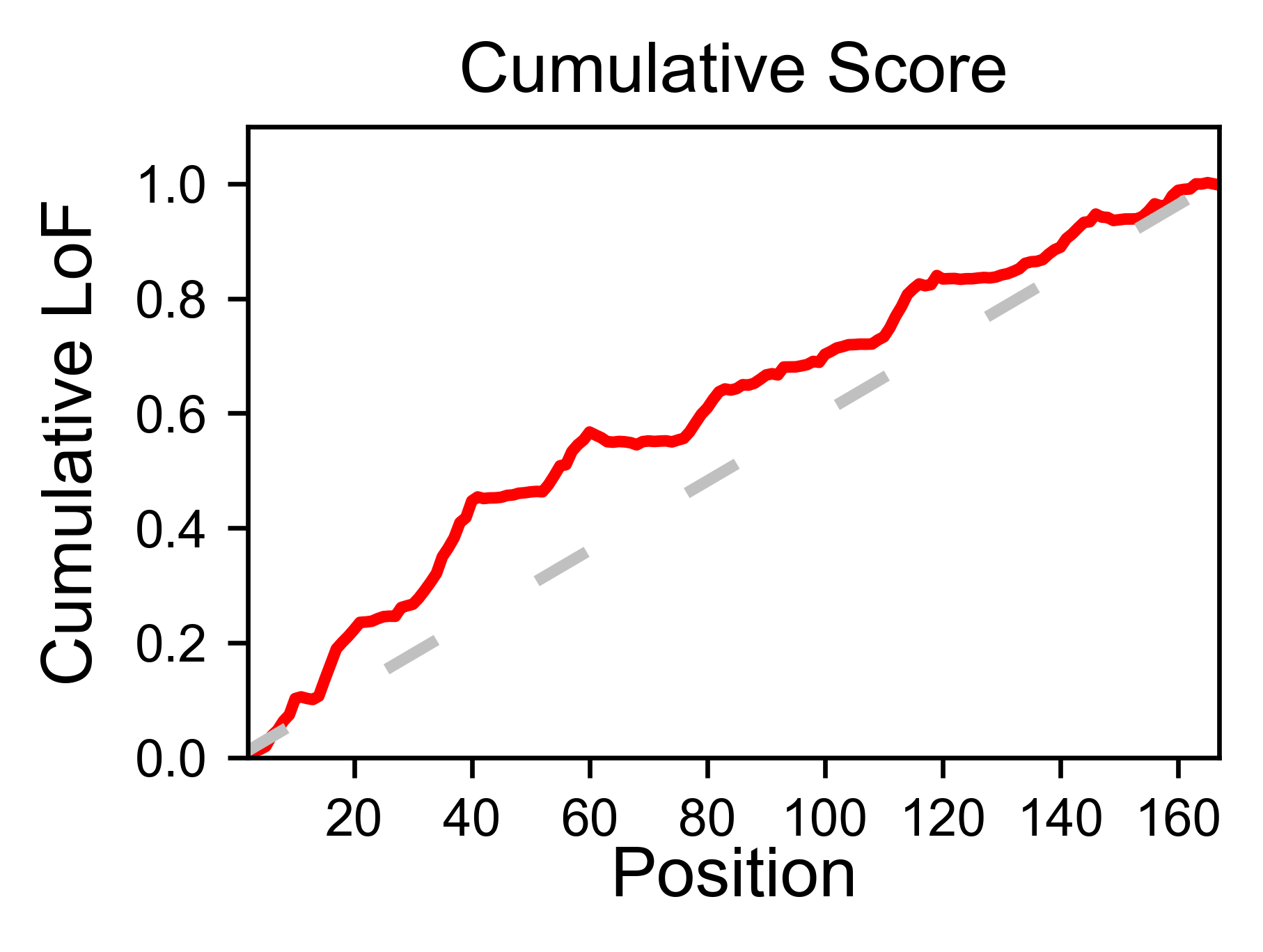## Bar and line charts¶

Classes reviewed in this section:

The method `object.enrichment_bar` will plot the mean enrichment score for every position on a bar chart. It will be colored blue for loss of function and red for gain of function. Additionally, setting the parameter `mode` to an amino acid (using the one letter code) will plot the enrichment for that particular amino acid along the protein. In this example, we are showing the mean enrichment scores (top) and an alanine scan (bottom)

```# Plot a bar graph with the mean enrichment score
hras_rbd.enrichment_bar(
figsize=[6, 2.5],
mode='mean',
show_cartoon=True,
yscale=[-2, 0.5],
title='',
)

# Plot a bar graph with the alanine enrichment score
hras_rbd.enrichment_bar(
figsize=[6, 2.5],
mode='A',
show_cartoon=True,
yscale=[-2, 0.5],
title='',
)
```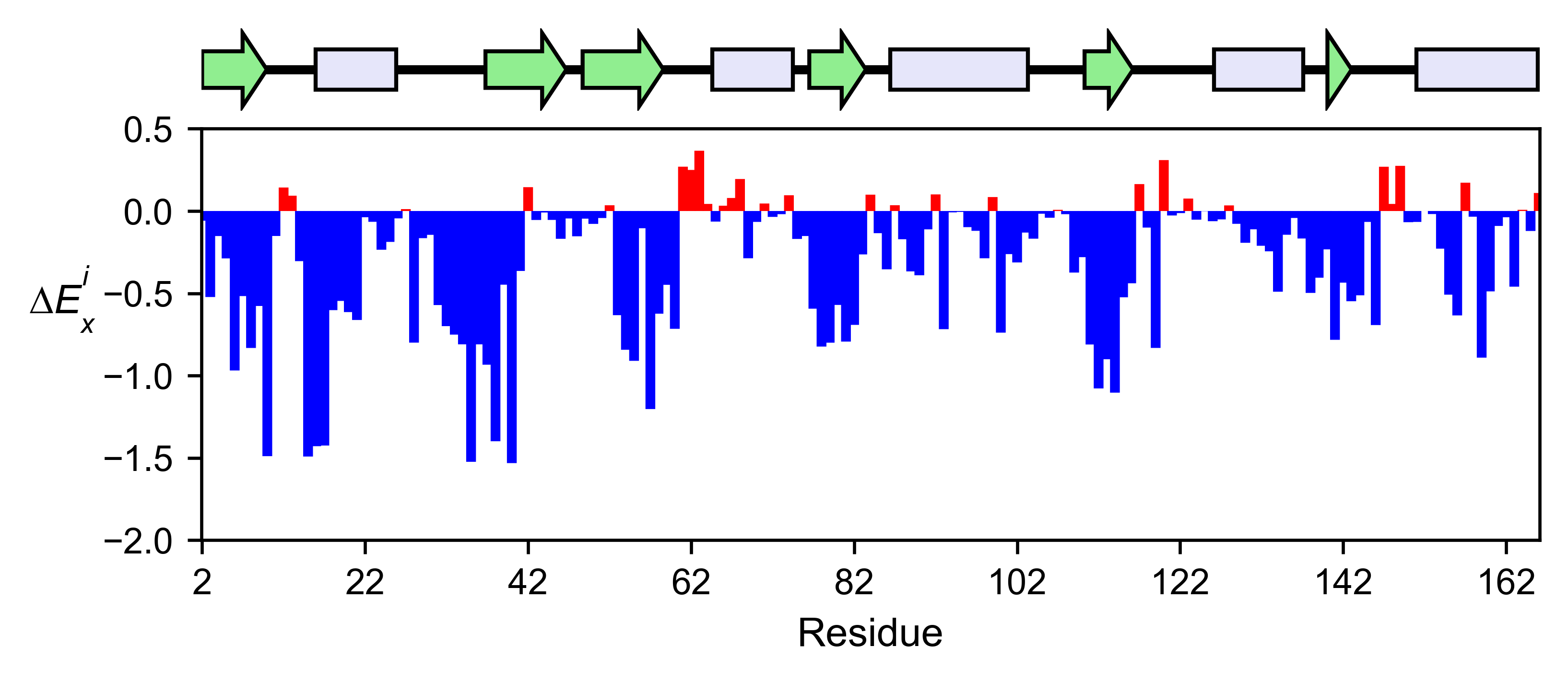The mean differential effect between the two example datasets is displayed using the method `object.differential`. This plot is useful to compare either orthologs/paralogs or the same protein with different effectors, and determine which areas of the protein have a different sensitivity to mutations.

```# Plot the difference between H-Ras unregulated and H-Ras regulated datasets
# The subtraction is hras_RBD - hrasGAPGEF
hras_rbd.differential(
hras_gapgef,
figsize=[6, 2.5],
show_cartoon=True,
yscale=[-1, 1],
title='',
)
```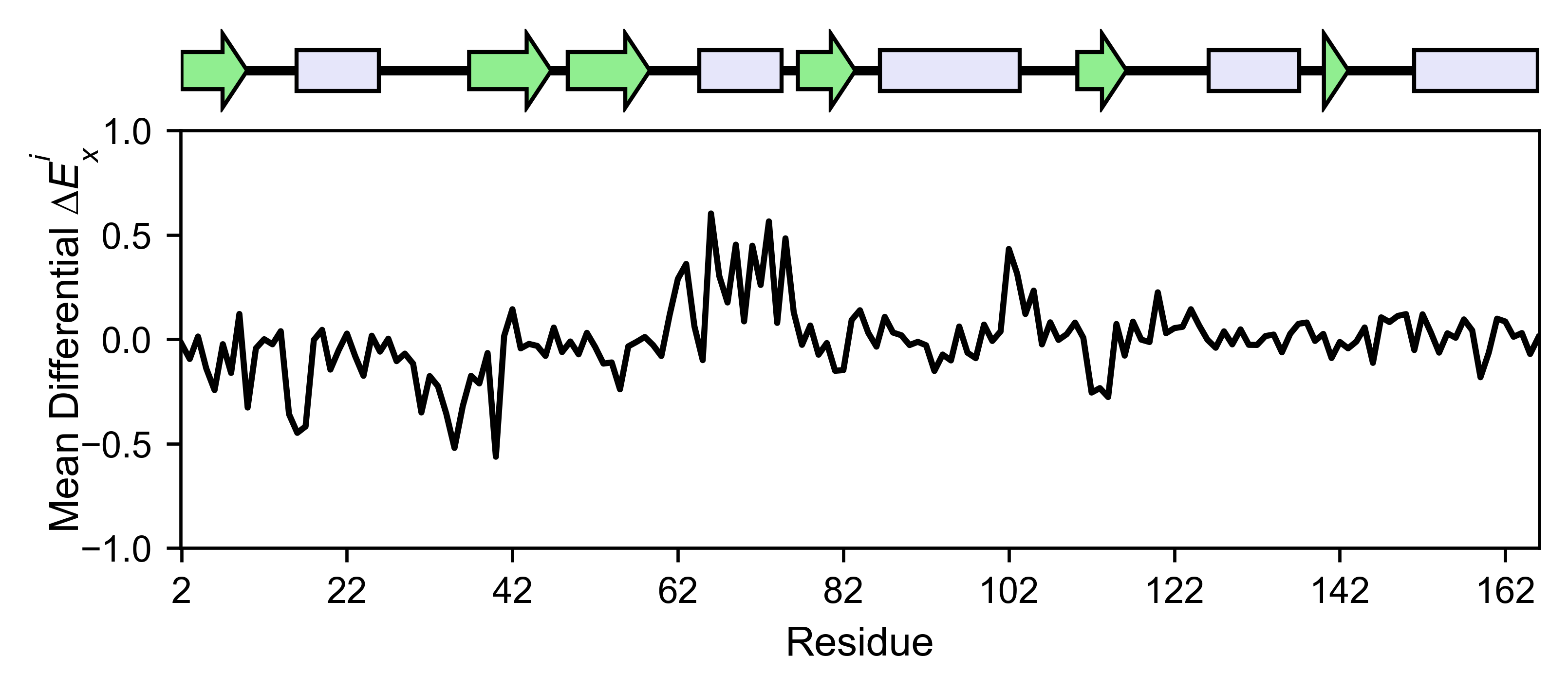You can check the individual mutational profile of a residue by using `object.position_bar`.

```# Create plot for position 117
hras_rbd.position_bar(
position=117,
yscale=(-1.5, 0.8),
figsize=(3.5, 2),
title='Position 117',
)
```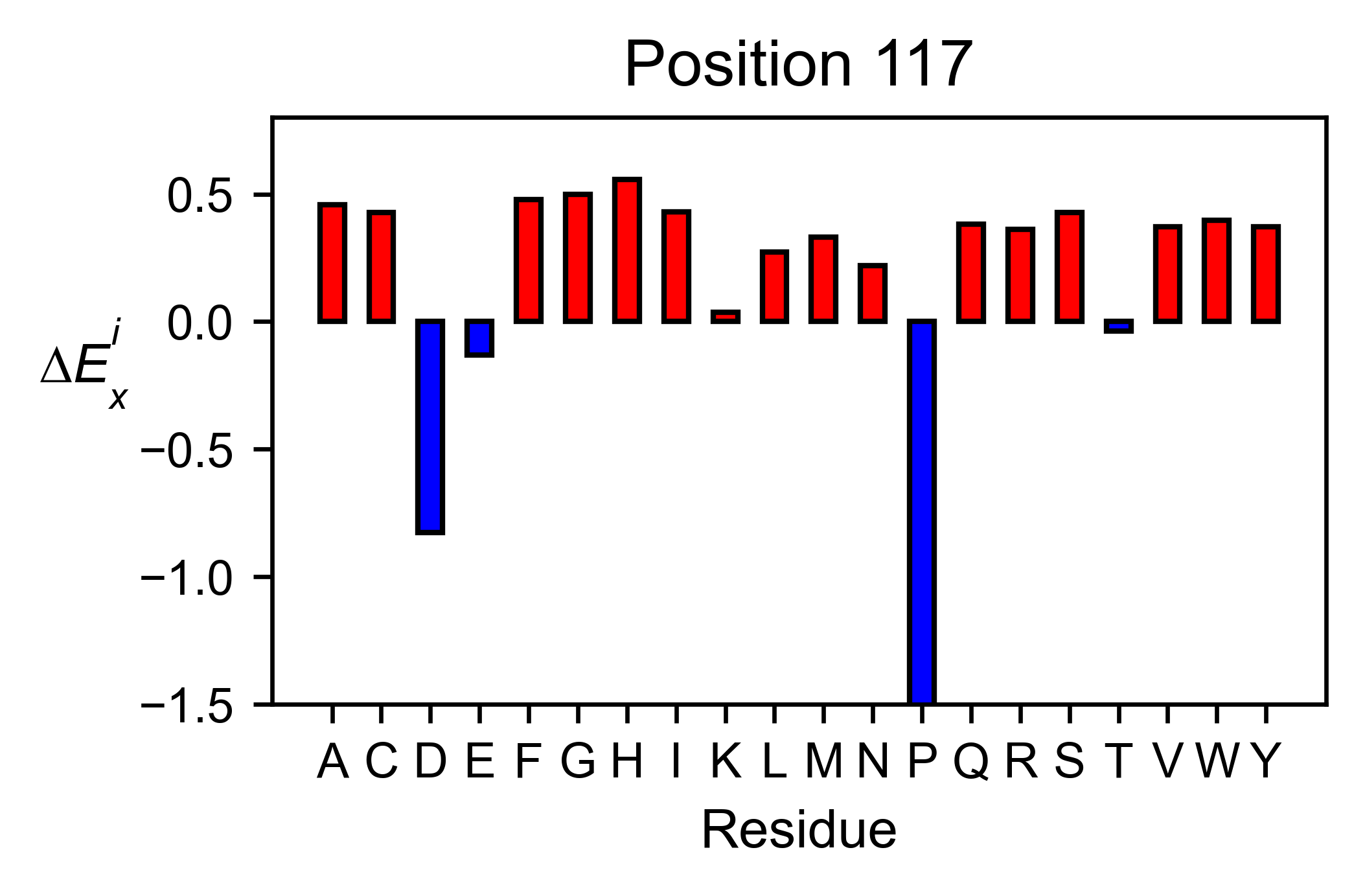If you added the secondary structure as an attribute of the object, you can plot the mean enrichment score for each alpha and beta motif in the protein (`object.secondary_mean`).

```# Graph bar of the mean of each secondary motif
hras_rbd.secondary_mean(
yscale=[-1, 0],
figsize=[3, 2],
title='Mean of secondary motifs',
output_file=None
)
```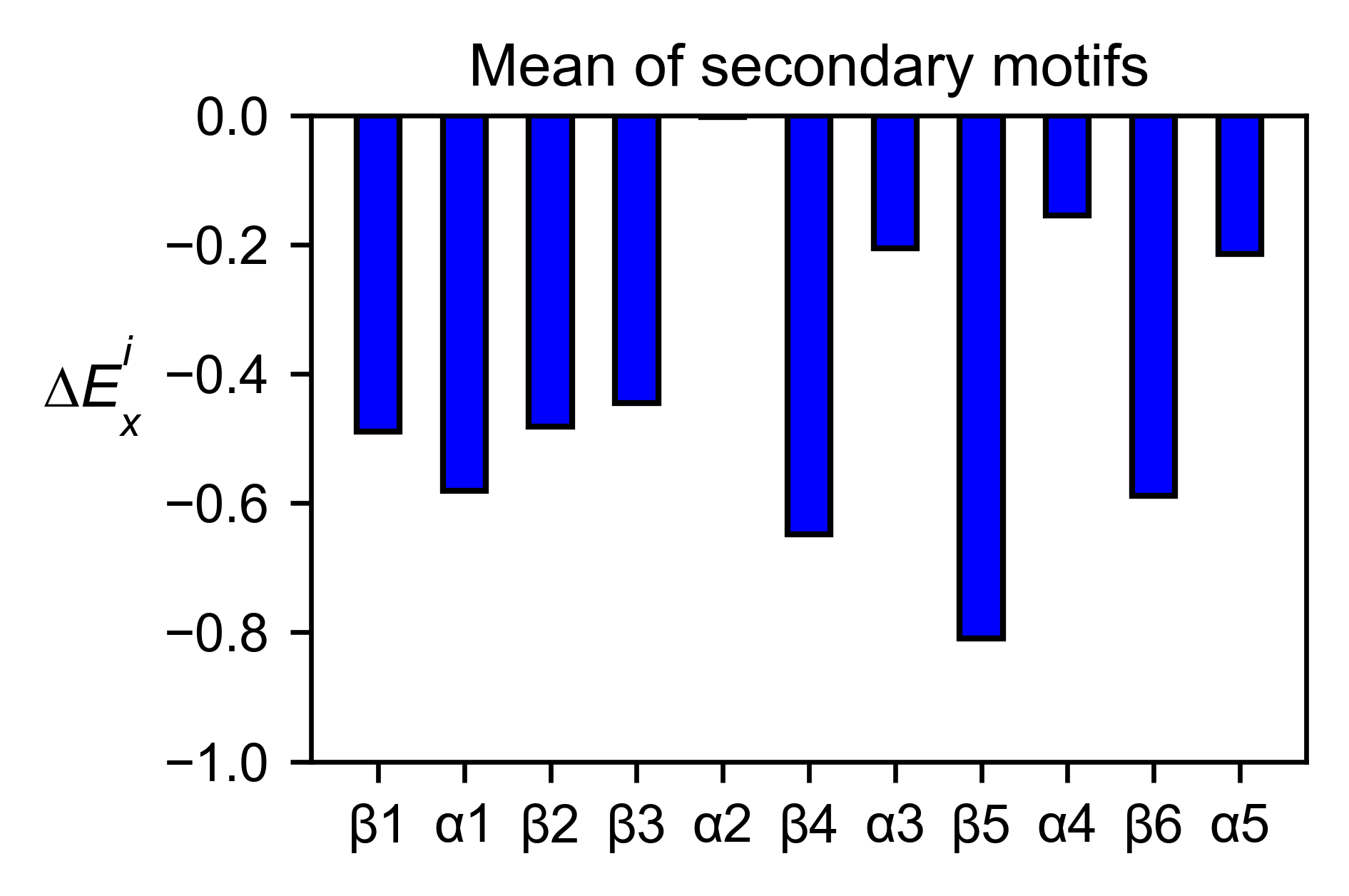## Correlation, PCA and ROC AUC¶

Classes reviewed in this section:

If you want to know more about PCA and ROC, watch the following StatQuest videos on youtube: PCA ROC and AUC

The correlation of amino acid substitution profiles can be calculated for each amino acid and graphed using `object.correlation`. In the example we observe that polar amino acids have high correlation between themselves but low correlation with hydrophobic amino acids.

```# Correlation between amino acids
hras_rbd.correlation(
colorbar_scale=[0.5, 1], title='Correlation'
)
```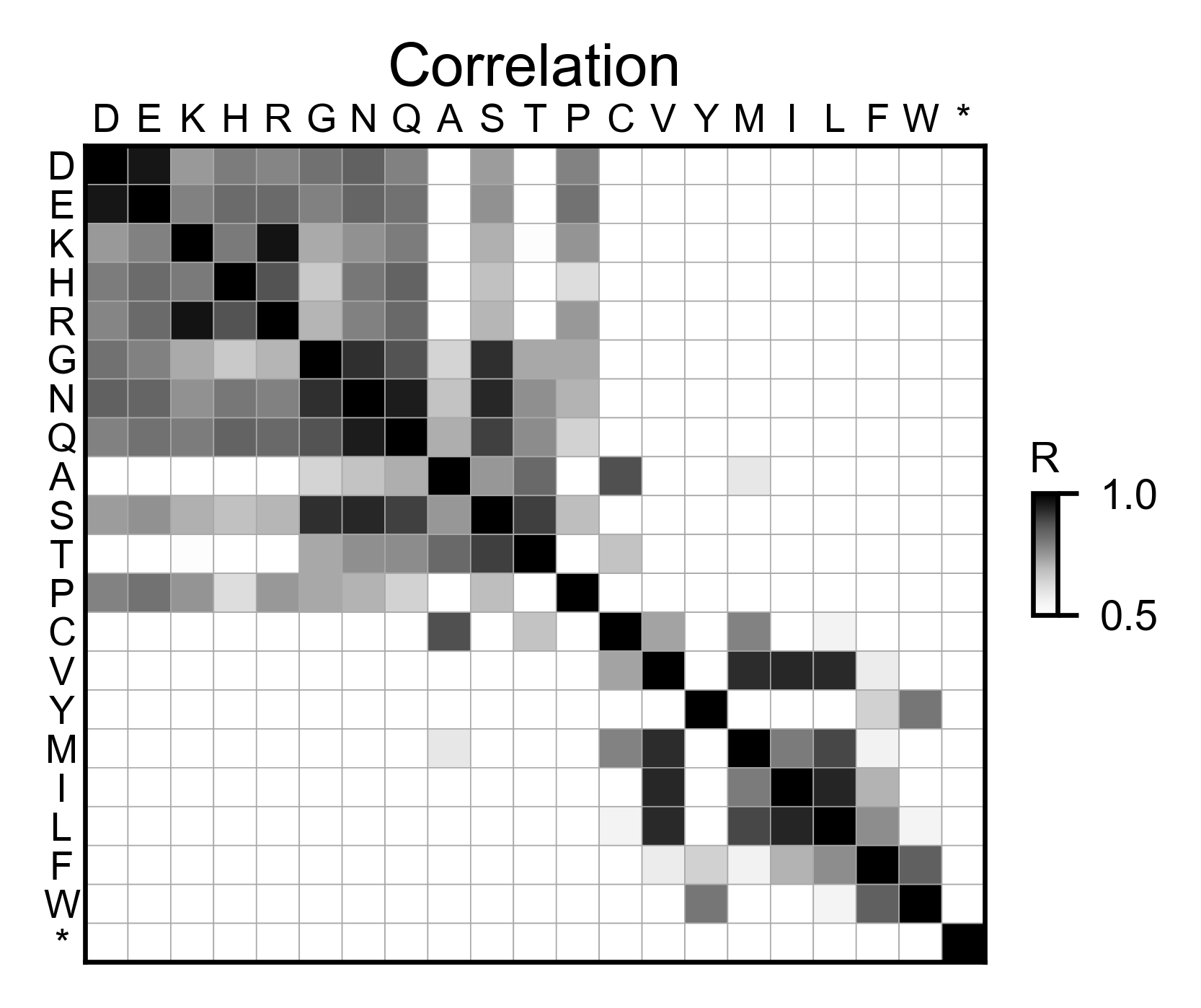The method `object.individual_correlation` will tell you how a single amino acid substitution profile (row of the heatmap) correlates to the rest of the dataset.

```# Explained variability by amino acid
hras_rbd.individual_correlation(
yscale=[0, 0.6],
title='Explained variability by amino acid',
output_file=None
)
```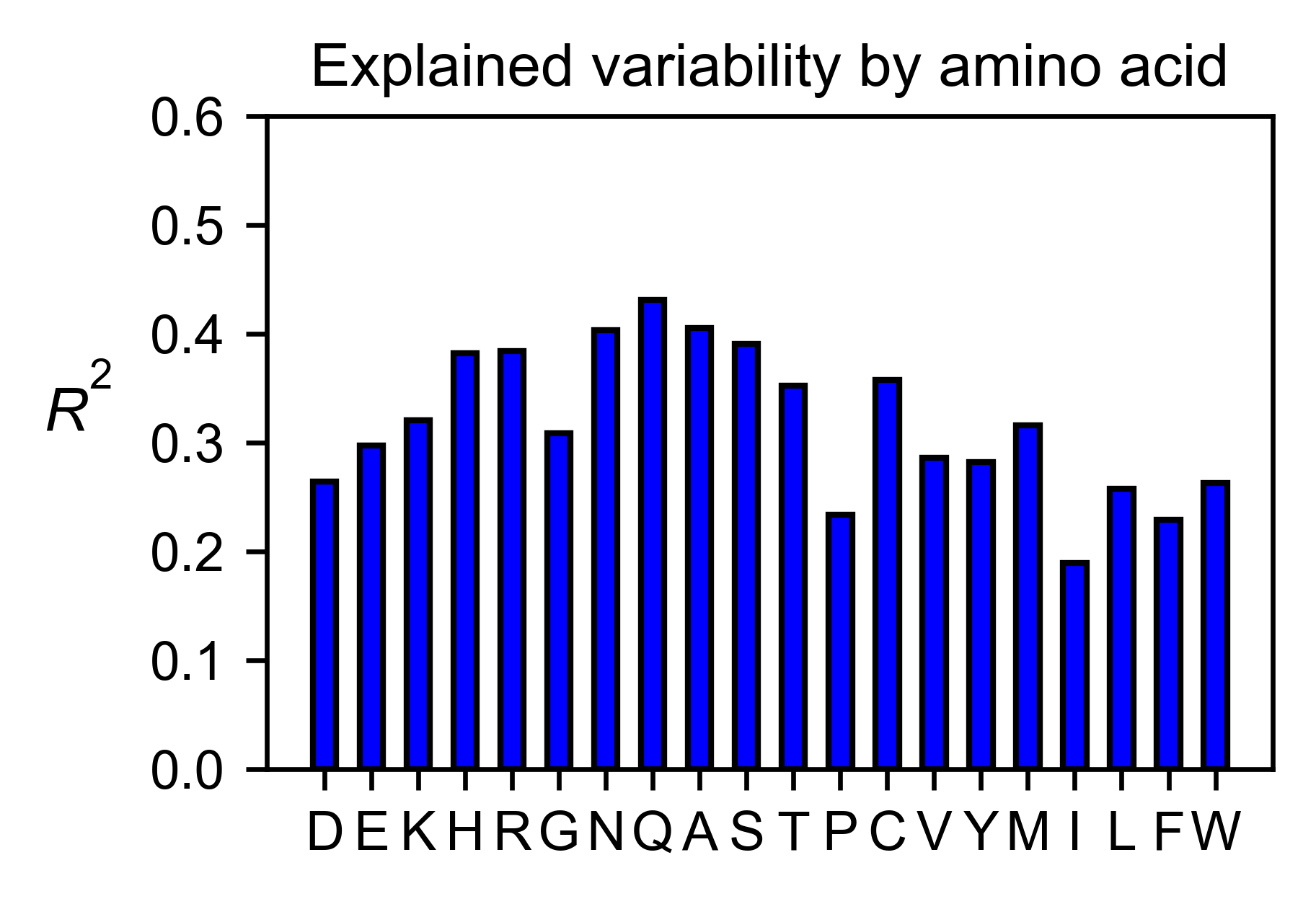The package can perform principal component analysis (PCA) using the method `object.pca`. The parameter `mode` can be set to `aminoacid`, in which will cluster amino acids based on their similarity, `individual` in which will do the same for each individual residue and `secondary`, in which will cluster for each motif. By default, the first two dimensions will be plotted (0 and 1 in Python notation), but that can be changed by `dimensions` parameter.

```# PCA by amino acid substitution
hras_rbd.pca(
title='',
dimensions=[0, 1],
figsize=(2, 2),
output_file=None
)

# PCA by secondary structure motif
hras_rbd.pca(
title='',
mode='secondary',
dimensions=[0, 1],
figsize=(2, 2),
output_file=None
)

# PCA by each individual residue. Don't set adjustlabels = True unless really big figsize
hras_rbd.pca(
title='',
mode='individual',
dimensions=[0, 1],
figsize=(5, 5),
output_file=None
)
```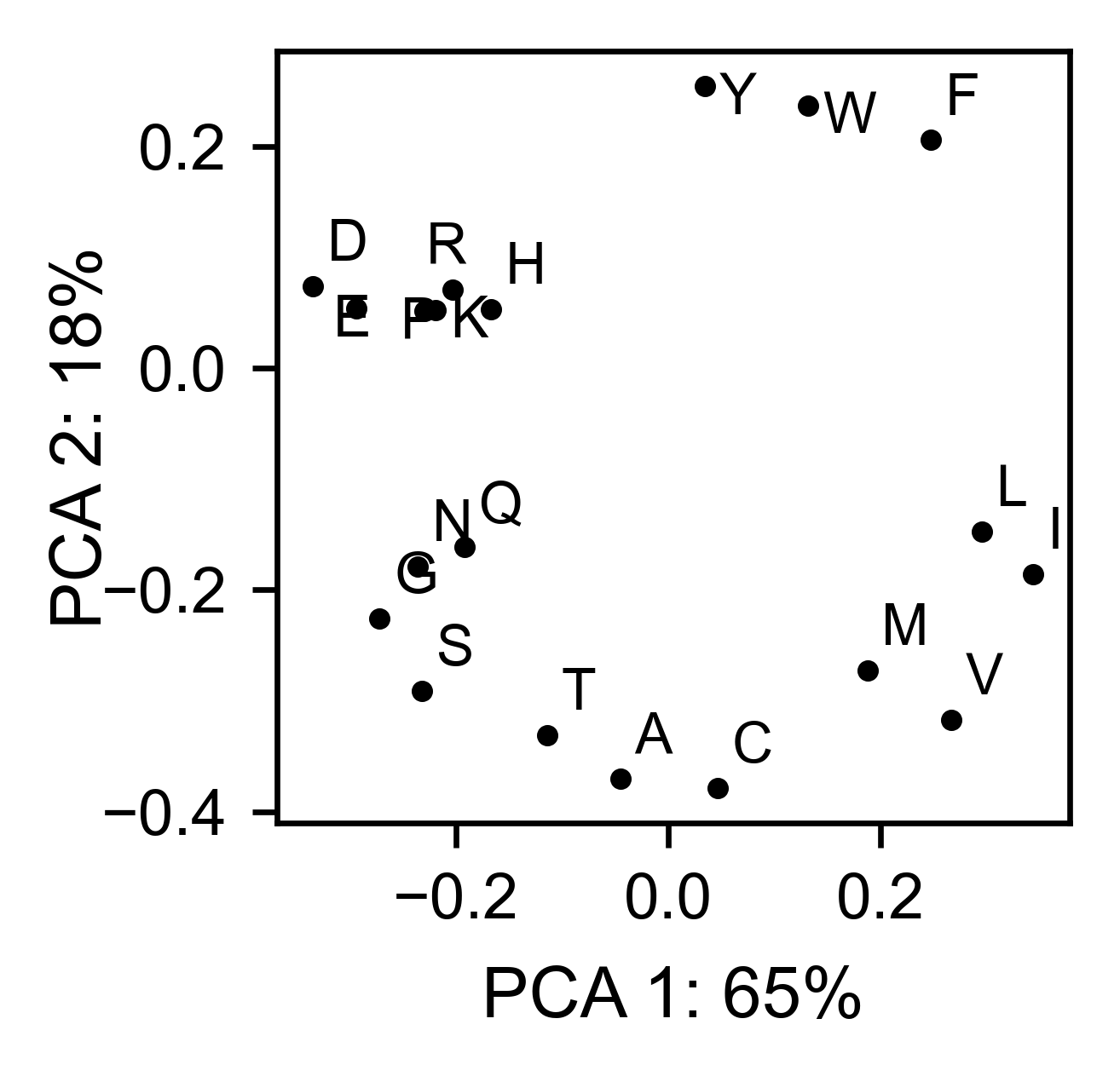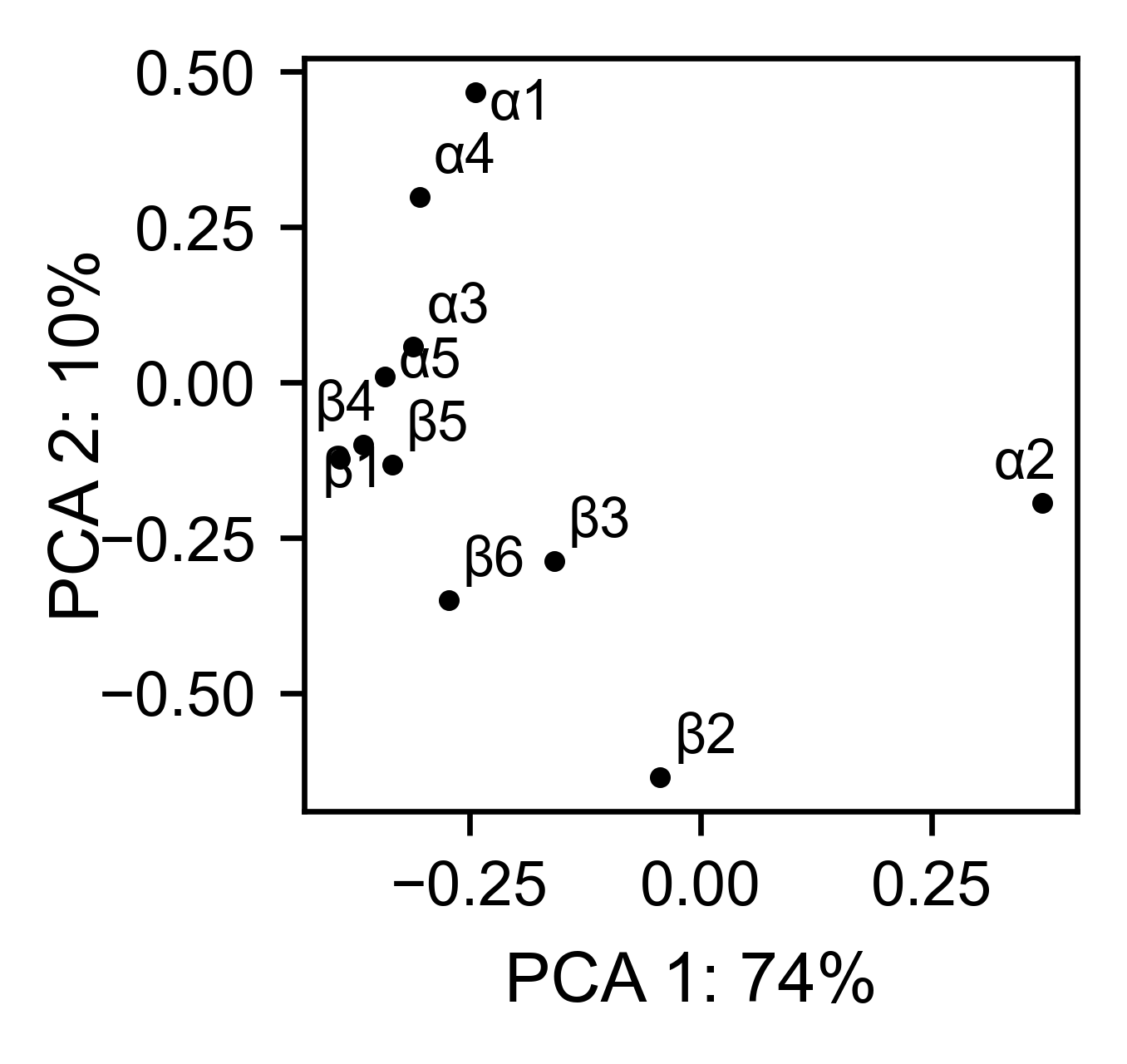Another type of plot that can be done is a receiver operating characteristic (ROC) curve for classification. You will use the method `object.roc` and as an input you will pass a dataframe that contains the label for each variant.

```#  Fake data
df_freq: DataFrame = DataFrame()
df_freq['Variant'] = hras_rbd.dataframes.df_notstopcodons[-1]['Variant']
df_freq['Class'] = np.random.randint(2, size=len(df_freq))

# Plot ROC curve
hras_rbd.roc(
df_freq[['Variant', 'Class']],
title='ROC example',
)
```## Pymol¶

Class reviewed in this section:

The data can be visualized on a Pymol object using `object.pymol`. It is important that not only Pymol is installed, but also on the same path as Python. You may have to manually install the ipymol API. See the Getting Started chapter for more information.

The parameter `pdb` will fetch the pdb that you want to use. Note that the protein chain needs to be specified (see example). Red for gain of function and blue for loss of function. `mode` lets you specifiy whether to plot the mean or an individual amino acid profile (left - Leucine, right - Aspartate).

```# Start pymol and color residues. Cut offs are set with gof and lof parameters.
hras_rbd.pymol(pdb='5p21_A', mode='mean', gof=0.2, lof=-0.5)

# Now check the mutational profile of Leucine (left image)
hras_rbd.pymol(pdb='5p21_A', mode='L', gof=0.2, lof=-0.5)

# Now check the mutational profile of Aspartate (right image)
hras_rbd.pymol(pdb='5p21_A', mode='D', gof=0.2, lof=-0.5)
```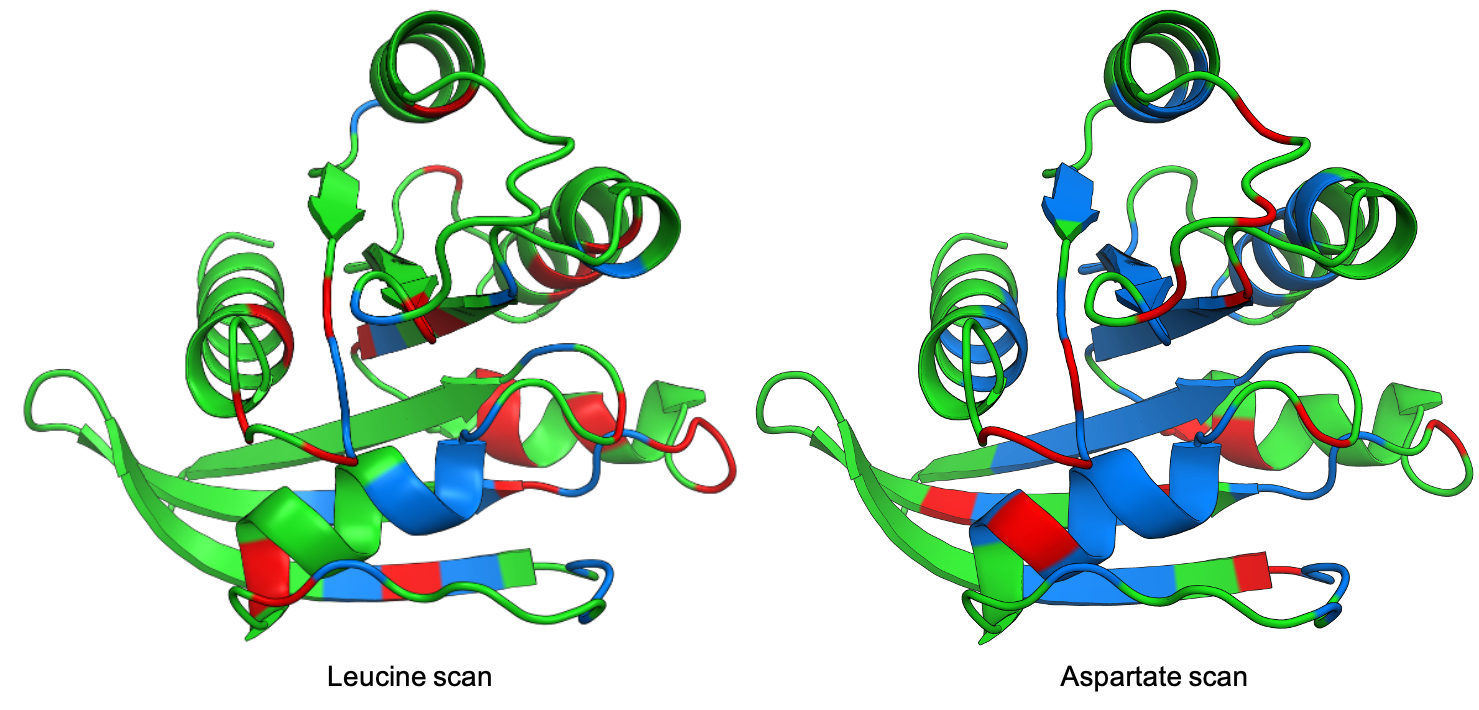## Art¶

The heatmap method can be used to generate artistic plots such as the one in the documentation overview. In here we show how that is done. On an Excel we have defined the color for each square in the heatmap (also available with the package, see `logo.xlsx`). The first step is to import the excel file, and then we perform the same steps as in a normal dataset.

```%matplotlib inline

from mutagenesis_visualization.main.classes.screen import Screen
from mutagenesis_visualization.main.utils.data_paths import PATH_LOGO
usecols = 'A:BL'
#df_logo = pd.read_excel(path, 'logo', usecols=usecols, nrows=21)
df_logo = pd.read_excel(PATH_LOGO, 'logo_2', usecols=usecols, nrows=21)

# Combine two dataframes
df_mixed = df_logo * 1.2 - df_faded

# Aminoacids
aminoacids = list('ACDEFGHIKLMNPQRSTVWY*')

# Define protein sequence
sequence_logo = "FUNNY THAT YOU ARE READING THIS SEQUENCE. NO SECRET CODE FOR NOW"

# Define secondary structure
secondary = [['L0'] * 5, ['β1'] * (9 - 1), ['L1'] * (15 - 9),
['α1'] * (25 - 20), ['L2'] * (32 - 25), ['β2'] * (42 - 32),
['L3'] * (50 - 42), ['α2'] * (58 - 50), ['L4'] * (70 - 58)]

# Create object
logo_obj = Screen(
df_mixed, sequence_logo, aminoacids = aminoacids, start_position=1, fillna=0, secondary=secondary
)

# Create hetmap
logo_obj.heatmap(
show_cartoon=True,
title='',
neworder_aminoacids='ACDEFGHIKLMNPQRSTVWY*',
)
```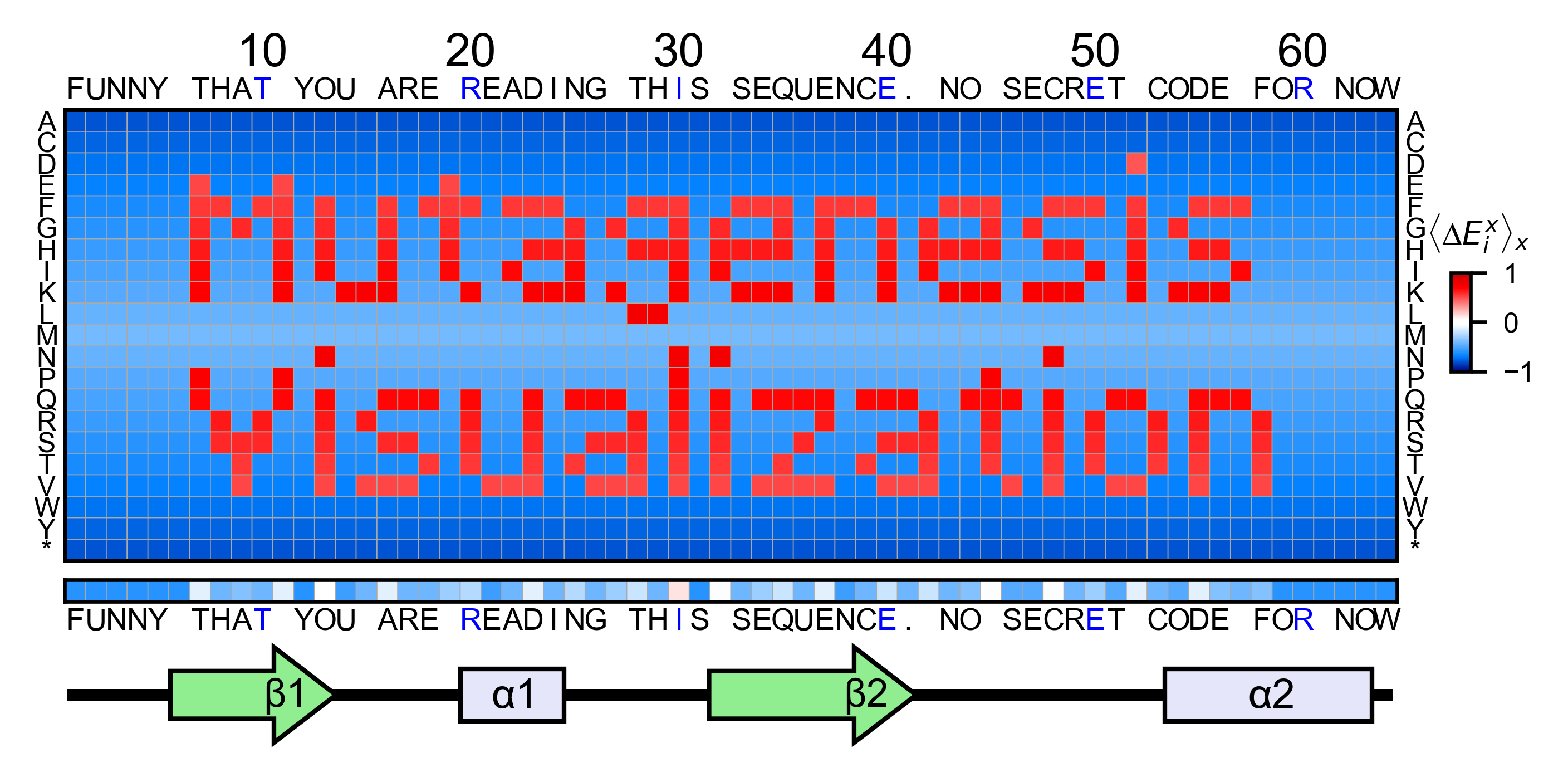## Reference¶

  Bandaru, P., Shah, N. H., Bhattacharyya, M., Barton, J. P., Kondo, Y., Cofsky, J. C., … Kuriyan, J. (2017). Deconstruction of the Ras switching cycle through saturation mutagenesis. ELife, 6. DOI: 10.7554/eLife.27810